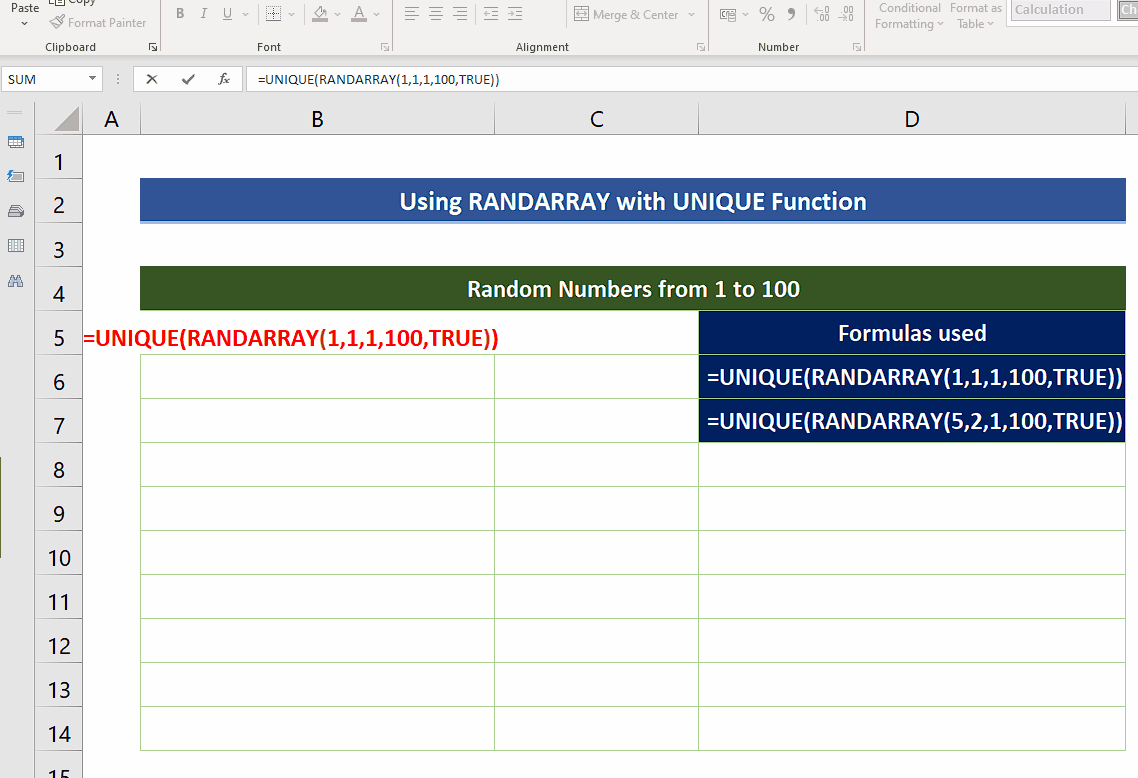# How to generate random numbers without repetition in Excel

In today’s tutorial we’ll learn how to generate random numbers from 1 to 100 without any repeats or duplicates. We’ll use the following functions of Excel one by one.

• UNIQUE with RANDARRAYExcel provides us with a variety of tools and functions to perform any type of calculation on the data set available. We can even generate sequential or non-sequential random numbers using built-in functions of Excel.

### Step 1 – Use RANDARRAY with UNIQUE to generate random number between 1 and 100 without duplicates– The RANDARRAY function returns an array of random numbers. You can specify the number of rows and columns to fill, minimum and maximum values, and whether to return whole numbers or decimal values. The syntax is as follows;
=RANDARRAY([rows], [columns], [min], [max], [whole_number])

– The important thing to keep in mind while filling in the values of minimum and maximum is that RANDARRAY generates the random numbers including the upper and lower boundaries. So, we’ll have to use the following formula to generate random numbers from 1 to 100.
=UNIQUE(RANDARRAY(1,1,1,100,TRUE))

– The current formula will generate only 1 row and 1 column, i.e., only one number with 1 as minimum value and 100 as maximum value. Also choose TRUE for getting integer values.

– If we want to generate the array of random numbers then change the values of rows and columns in the RANDARRAY function as per your requirement or use the formula mentioned below to generate an array of 5 rows and 2 columns.
=UNIQUE(RANDARRAY(5,2,1,100,TRUE))